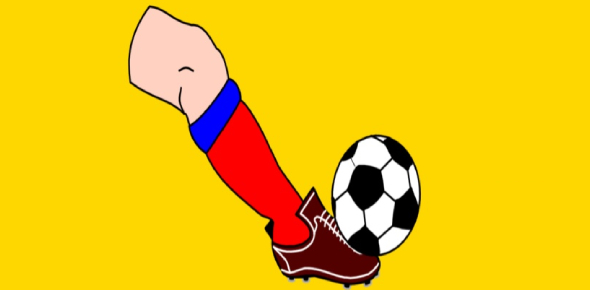# Motion And Force Basic Concepts: Quiz!

10 Questions | Attempts: 2213
ShareSettingsA force is a pushing or pulling movement that can make things move or change direction. In contrast, motion is the displacement of a body from an initial point. This quiz has been created to test your knowledge about basic concepts related to force and motion. Let's try it out!

• 1.
Which tools are needed to measure the speed of a rolling soccer ball?
• A.

Thermometer, stopwatch

• B.

Thermometer, balance

• C.

Spring scale, ruler

• D.

Stopwatch, ruler

• 2.
Which of the following is most likely to increase friction?
• A.

Grooved rubber soles on shoes

• B.

Wheels on roller skates

• C.

• D.

Wax on wood floors

• 3.
The force that pulls objects to the ground on Earth is known as__.
• A.

Magnetism

• B.

Collision

• C.

Friction

• D.

Gravity

• 4.
Which of the following is a non-contact force?
• A.

Collision

• B.

Friction

• C.

Magnetism

• D.

None of the above

• 5.
Without ___, we would not be able to walk or climb stairs.
• A.

Collision

• B.

Magnetism

• C.

Friction

• D.

Gravity

• 6.
A push or a pull on an object is:
• A.

A force

• B.

Inertia

• C.

Its weight

• D.

Motion

• 7.
Forces can change the:
• A.

Slow down or speed up objects only

• B.

Slow down or speed up objects only

• C.

Movement of objects but only if they were already in motion

• D.

None

• 8.
Balanced forces act on objects that are:
• A.

Stationary

• B.

In motion

• C.

Rising or falling

• D.

None

• 9.
Sir Isaac Newton stated that for every action, there was:
• A.

An equal and opposite reaction

• B.

Movement in a direction that was the same as the motion

• C.

A force of gravity also acting

• 10.
The size or magnitude of a frictional force depends on:
• A.

The forces pushing the surfaces together

• B.

The roughness of the contact surfaces

• C.

Both of the above

• D.

None

## Related TopicsBack to top
×

Wait!
Here's an interesting quiz for you.